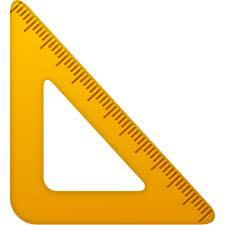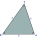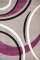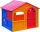# Right Δ

A right triangle has the length of one leg 11 cm and length of the hypotenuse 61 cm. Calculate the height of the triangle.

Result

vc =  10.8 cm

#### Solution:

$a = 11 \ cm \ \\ c = 61 \ cm \ \\ b = \sqrt{ c^2-a^2 } = 60 \ cm \ \\ \ \\ S = S \ \\ \dfrac{1}{2} c v_c = \dfrac{1}{2} a b \ \\ c v_c = a b \ \\ c v_c = a v_c = \dfrac{a}{c} b = \dfrac{ 11}{ 61} \cdot 60 = 10.8 \ \text{cm}$

Try calculation via our triangle calculator.Our examples were largely sent or created by pupils and students themselves. Therefore, we would be pleased if you could send us any errors you found, spelling mistakes, or rephasing the example. Thank you!

Leave us a comment of this math problem and its solution (i.e. if it is still somewhat unclear...):

Showing 1 comment:Math student
Maths

1 year ago  1 LikeTips to related online calculators
Do you have a linear equation or system of equations and looking for its solution? Or do you have quadratic equation?
Pythagorean theorem is the base for the right triangle calculator.
Do you want to convert length units?

#### You need to know the following knowledge to solve this word math problem:

We encourage you to watch this tutorial video on this math problem:

## Next similar math problems:

1. The right triangleThe right triangle ABC has a leg a = 36 cm and an area S = 540 cm2. Calculate the length of the leg b and the median t2 to side b.
2. Triangular prismThe base of the perpendicular triangular prism is a rectangular triangle with a hypotenuse of 10 cm and one leg of 8 cm. The prism height is 75% of the perimeter of the base. Calculate the volume and surface of the prism.
3. The basesThe bases of the isosceles trapezoid ABCD have lengths of 10 cm and 6 cm. Its arms form an angle α = 50˚ with a longer base. Calculate the circumference and content of the ABCD trapezoid.
4. Horses playgroundThe fence for the horses has the shape of a rectangular trapezoid with an area of 400 m2, the base lengths should be 31 m and 19 m. How many meters of boards will they need to fence it if the boards are stacked in 5 rows?
5. Triangular prism - regularThe regular triangular prism is 7 cm high. Its base is an equilateral triangle whose height is 3 cm. Calculate the surface and volume of this prism.
6. Annulus from triangleCalculate the content of the area bounded by a circle circumscribed and a circle inscribed by a triangle with sides a = 25mm, b = 29mm, c = 36mm
7. An equilateralAn equilateral triangle is inscribed in a square of side 1 unit long so that it has one common vertex with the square. What is the area of the inscribed triangle?
8. FlakesA circle was described on the square, and a semicircle above each side of the square was described. This created 4 "flakes". Which is bigger: the content of the central square or the content of four chips?
9. Height to the baseThe triangle area is 35 cm ^ 2. The size of the base is 10 cm. Find the length of height to the base.
10. Compute 4Compute the exact value of the area of the triangle with sides 14 mi, 12 mi, and 12 mi long.
11. Squares ratioThe first square has a side length of a = 6 cm. The second square has a circumference of 6 dm. Calculate the proportions of the perimeters and the proportions of the contents of these squares? (Write the ratio in the basic form). (Perimeter = 4 * a, conte
12. A mapA map with a scale of 1: 5,000 shows a rectangular field with an area of 18 ha. The length of the field is three times its width. The area of the field on the map is 72 cm square. What is the actual length and width of the field?
13. The hollow cylinderThe hollow cylinder has a height of 70 cm, an outer diameter of 180 cm and an inner diameter of 120 cm. What is the surface of the body, including the area inside the cavity?
14. Wallpaper3750 cm square of wallpaper is needed to glue a cube-shaped box. Can Dad cut out the whole necessary piece of wallpaper as a whole if he has a roll of wallpaper 50 cm wide?
15. Garden exchangeThe garden has the shape of a rectangular trapezoid, the bases of which have dimensions of 60 m and 30 m and a vertical arm of 40 m. The owner exchanged this garden for a parallelogram, the area of which is 7/9 of the area of a trapezoidal garden. What is
16. CarpetHow many crowns CZK do we pay for a carpet for a bedroom, when 1m of square carpet costs 350 CZK and the bedroom has dimensions of 4m and 6m? How many crowns do we pay for a strip around the carpet, when 1m of the strip costs 15 CZK?
17. Rectangular landOn a rectangular land with dimensions of 35 m and 18.5 m is a house with a square floor plan with a side of 14 m. What % of the land is not occupied?